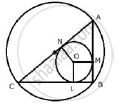Share

Prove that the Perimeter of a Right Triangle is Equal to the Sum of the Diameter of Its Incircle and Twice the Diameter of Its Circumcircle. - ICSE Class 10 - Mathematics

ConceptArc and Chord Properties - Angle in a Semi-circle is a Right Angle

Question

Prove that the perimeter of a right triangle is equal to the sum of the diameter of its incircle and twice the diameter of its circumcircle.

SolutionJoin OL, OM and ON.
Let D and d be the diameter of the circumcircle and incircle.
and let R and r be the radius of the circumcircle and incircle.
In circumcircle of  ΔABC,
∠B = 90
Therefore, AC is the diameter of the circumcircle i.e. AC = D
Let radius of the incircle = r
∴ OL =  OM = ON = r
Now, from B, BL, BM are the tangents to the incircle.
∴ BL = BM = r
Similarly,
AM = AN and CL = CN = R
(Tangents from the point outside the circle)
Now,

AB + BC +CA = AM + BM +BL +CL +CA

= AN + r + r + CN + CA

= AN + CN + 2r + CA

= AC + AC + 2r

= 2AC + 2r

=2D + d

Is there an error in this question or solution?

Video TutorialsVIEW ALL 

Solution Prove that the Perimeter of a Right Triangle is Equal to the Sum of the Diameter of Its Incircle and Twice the Diameter of Its Circumcircle. Concept: Arc and Chord Properties - Angle in a Semi-circle is a Right Angle.
S